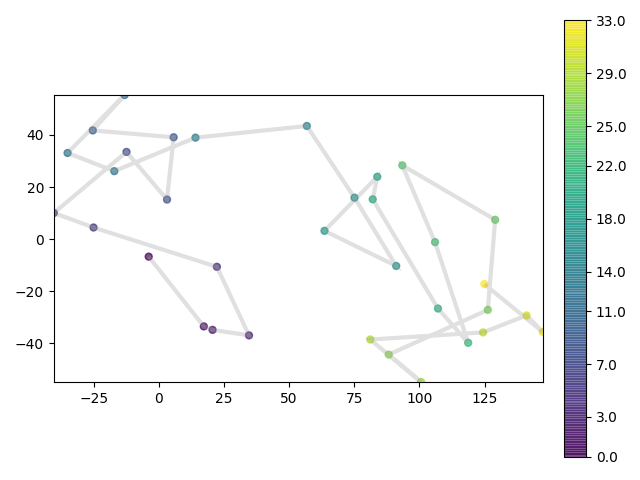# Pandas Indexing and Resampling¶

Traja is built on top of pandas `DataFrame`, giving access to low-level pandas indexing functions.

This allows indexing, resampling, etc., just as in pandas:

```from traja import generate, plot
import pandas as pd

# Generate random walk
df = generate(n=1000, fps=30)

# Select every second row
df[::2]

Output:
x         y      time
0  0.000000  0.000000  0.000000
2  2.364589  3.553398  0.066667
4  0.543251  6.347378  0.133333
6 -3.307575  5.404562  0.200000
8 -6.697132  3.819403  0.266667
```

You can also do resampling to select average coordinate every second, for example:

```# Convert 'time' column to timedelta
df.time = pd.to_timedelta(df.time, unit='s')
df = df.set_index('time')

# Resample with average for every second
resampled = df.resample('S').mean()
plot(resampled)
```